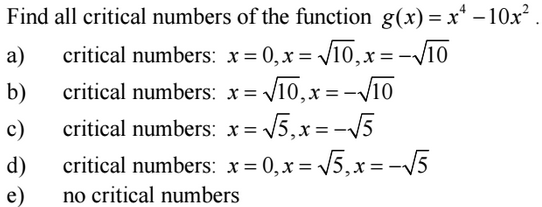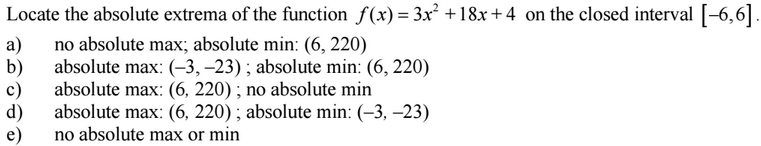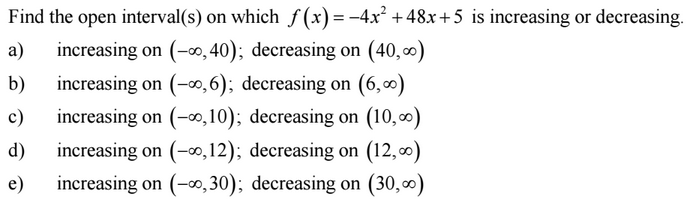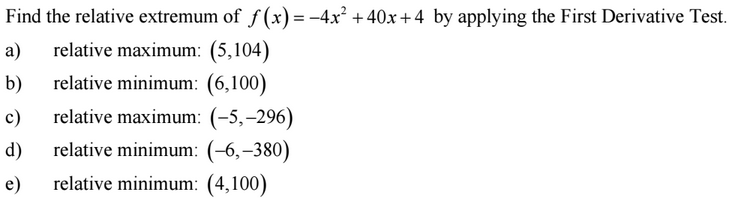### Day 37 - Rolle's Theorem and Mean Value Theorem - 10.07.15

Update
• Summative Exam 1
• Average = 75%

Bell Ringer

1. Critical Numbers1. Extrema1. Increasing/Decreasing Functions1. Relative ExtremaReview
• Pre-calculus
• Extrema
• Minima
• Maxima
• Absolute
• Relative
• Interval Notation
• Extrema (video) (checkpoints)
• How can extrema be defined for a function?
• How can critical numbers be calculated using derivatives?
• How are critical numbers related to extrema?
• Absolute and Relative Extrema (video)
• Critical Numbers (video)
• Increasing/Decreasing Functions (video) (checkpoints)
• How are derivatives related to functions increasing and decreasing?

Lesson
• Rolle's Theorem
• Mean Value Theorem
• Objective
• How are Rolle's Theorem and Mean Value Theorem related to differentiation?
• Challenge 12 (no computers allowed)
• Videos
• Practice

Exit Ticket
• Posted on the board at the end of the block (link).

Homework
• WNQ the following videos:

#### In-Class Help Requests

Standard(s)
• APC.7
• Analyze the derivative of a function as a function in itself.
• Includes:
• comparing corresponding characteristics of the graphs of f, f', and f''
• ​defining the relationship between the increasing and decreasing behavior of f and the sign of f'
• ​translating verbal descriptions into equations involving derivatives and vice versa
• analyzing the geometric consequences of the Mean Value Theorem;
• defining the relationship between the concavity of f and the sign of f"; and ​identifying points of inflection as places where concavity changes and finding points of inflection.
• APC.8
• Apply the derivative to solve problems.
• Includes:
• ​analysis of curves and the ideas of concavity and monotonicity
• optimization involving global and local extrema;
• modeling of rates of change and related rates;
• use of implicit differentiation to find the derivative of an inverse function;
• interpretation of the derivative as a rate of change in applied contexts, including velocity, speed, and acceleration; and
• differentiation of nonlogarithmic functions, using the technique of logarithmic differentiation.*
• *AP Calculus BC will also apply the derivative to solve problems.
• Includes:
• ​analysis of planar curves given in parametric form, polar form, and vector form, including velocity and acceleration vectors;
• ​numerical solution of differential equations, using Euler’s method;
• ​l’Hopital’s Rule to test the convergence of improper integrals and series; and
• ​geometric interpretation of differential equations via slope fields and the relationship between slope fields and the solution curves for the differential equations.